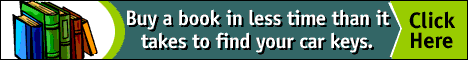Abstract. Keywords list for contributions to the Nexus Network Journal, including the copyright policy and information disclosure policy.

#Architecture and Mathematics Keywords for Contributions to the NNJCompiled by Stephen R. Wassell

Architecture Keywords:

accoustics;
allegory;
anthropomorphism;
building type (specify);
design analysis
design theory
didactics;
geographical area (specify);

historical era (specify);
historical treatise
individual architect (specify);
individual monument (specify);
landscape architecture;
meaning;
music theory;
myth;
optics;

ornament;
perspective;
representations of architecture (paintings, etc);

structural systems;
symbolism;

urban design;

Mathematics Keywords

algebra
algorithms
anthyphairesis
Archimedean solids (if specific semi-regular polyhedron, specify)
arithmetic mean
arithmetic progressions
automation
cabala (cabbala, cabbalah, kabala, kabbala, kabbalah)
calculus
chaos
circles
combinatorics
commensurables / commensurable ratios
computer science
computer technology
cones
conics / conic sections
convergence
cubic equations
deduction
descriptive geometry
diagonals
didactics
differential equations
dimensions (first, second, third, fourth, etc.)
discrete mathematics
doubling the cube
dynamics
ellipses
ellipsoids
elliptic paraboloids
engineering
equilibrium / equilibria
Euclidean geometry
even and odd numbers
feedback
fractals
geometry
geometric analysis
geometric mean
geometric progressions
gnomons
golden section / golden number / golden mean
grids
groups / group theory
harmonic mean
harmonic progressions
harmonic proportions
history of mathematics (if specific mathematician, specify)
hyperbolas
hyperbolic paraboloids
hyperboloids
incommensurables / incommensurable ratios
induction
infinity
irrational numbers / irrationals
iteration
linear algebra
linear programming
lines
logarithms
logarithmic spirals
logarithmic rosettes
magic squares
mandalas
mappings (transformations)
matrix / matrices
means
measurement / measuring systems
mechanics
metaphysics
metrology
metallic means
minimal surfaces
modeling
modules
Modulor
morphology
non-Euclidean geometry
non-linear dynamics
number
number series
number symbolism
number theory
ovals
parabolas
paraboloids
patterns
perfect numbers
perspective
perspective geometry
philosophy
physics
plastic number
Platonic solids (if specific regular polyhedron, specify)
polygons
polyhedron / polyhedra
polytopes
prime numbers
progressions
projective geometry
proportion
proportional analysis
proportional systems
randomness
ratio
rational numbers / rationals
rational convergents
regular polygons (equilateral triangle, square, regular pentagon, etc.)
rosettes
rule-based architecture
sacred cut
sacred geometry
sequences (an unending list of numbers; also called "progressions")
series (a sum of numbers; often incorrectly used in place of "sequences")
shapes (if specific shape, specify)
shape grammars
silver mean
spheres
spirals
statics
statistics
structures / structural engineering
squaring the circle (quadrature of the circle)
symmetry
symmetry groups
tessellations / tilings
tetraktys
topography
topology
transformations
trigonometry
trigonometric analysis
vesica piscis / vesica pisces
virtual architecture
virtual realitytop of page

NNJ Homepage

Order Nexus books!

Reaseach Articles

Didactics

Geometer's Angle

Book and Article Reviews

Conference and Exhibit Reports